# ISEE Upper Level Quantitative : How to find the length of the side of a rhombus

## Example Questions

### Example Question #61 : Quadrilaterals

In Rhombus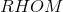,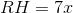and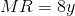. Which is the greater quantity?

(A)(B)(a) is the greater quantity

(b) is the greater quantity

It cannot be determined which of (a) and (b) is greater

(a) and (b) are equal

(a) is the greater quantity

Explanation:

The four sides of a rhombus, by defintion, have equal length, so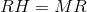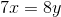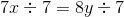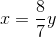Sinceandare positive,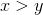.